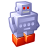# QlikView App Dev

Discussion Board for collaboration related to QlikView App Development.

cancel
Showing results for
Did you mean:Creator

## Conditional aggregation based on records before selection

Hi

Short Story:

I have some problems regarding the understanding the aggregation formula, in a case where I need to test if a customer has made any purchases before the selection; so I can divide sales in "new customers" and "Old Customers". If a customer make several purchases in the selected period; all the purchases should be counted as "New Customer"

Setting of issue:

I need to make this pivot that splits sales data by day based on the users date selections (From multiple "unknown" date fields in a Calendar Table);

E.g. Year, Month, Day, Week, Financial Year, YTD, WTD and so on.

Date:

20140101: 100

20140102: 75

20140103: 150

20140104: 25

Dimension: Date.

Expression: Sum(Sales)

So far so good.

My Issue:

My problem is to split the Sales Data into "new" and "old" customers based on customers, has made any purchases before the first selected date.

I have tried to make a Set Analysis that ignores all date Selections and inverts the selection to all days before the selections:

{1<[\$(=Concat({1<\$Table={'Calendar'}>} distinct \$Field,']=,[')&']='), Date={"<\$(=min(Date))"}>}

I have put this set analysis in a variable called \$(PrevDays) and my expression looks something like this:

Sum({\$<MemberGroup={1}, aggr(If(Sum(\$(vPrevDays) Sales)>0, Sum(Sales)), Member))

It does the test for previous purchases in the total; but puts all the following transaction in a day.

Customer 1 has made these sales in 2014:

20140101: 25

20140102: 0

20140103: 0

20140104: 20

And Customer 2 has made these sales in 2014:

20140101: 0

20140102: 10

20140103: 0

20140104: 0

My chart now shows the following:

20140101: 45

20140102: 10

20140103: 0

20140104: 0

Where I would like it to show something like this:

Old Customers:

20140101: 25

20140102: 10

20140103: 0

20140104: 25

New Customers:

20140101: 75

20140102: 60

20140103: 150

20140104: 5

Ps. If I use the "Aggr(NoDistinct" i gets this:

Old Customers

20140101: 45

20140102: 10

20140103: 0

20140104: 45

Hope someone would share their thoughts with me on this one - and please let me know if i need to explain more of the details.

Thanks

1 Solution

Accepted SolutionsCreator
Author

I think I have solved the issue.

I needed to use two aggr(-statements to allow the test to perform correct and then the data to show like i wanted:

My Expression is like following

Sum({\$<Type={1}>} aggr(if(Sum(aggr(NoDistinct Sum({\$<[\$(=Concat({1<\$Table={'Calendar'}>} distinct \$Field,']=,[')&']='), Date={"<\$(=min(Date))"}>}

Total <Customer> Sales), Customer))=0, Sum(Sales)), Date, Customer))

Or

Sum({\$<Type=1}>} aggr(if(Sum(aggr(NoDistinct Sum(\$(PrevDays) Total <Customer> Sales), Customer))=0, Sum(Sales)), Date, Customer))

Where \$(PrevDays) = {\$<[\$(=Concat({1<\$Table={'Calendar'}>} distinct \$Field,']=,[')&']='), Date={"<\$(=min(Date))"}>}

Hope other can find use of this case in futureCreator
Author

I think I have solved the issue.

I needed to use two aggr(-statements to allow the test to perform correct and then the data to show like i wanted:

My Expression is like following

Sum({\$<Type={1}>} aggr(if(Sum(aggr(NoDistinct Sum({\$<[\$(=Concat({1<\$Table={'Calendar'}>} distinct \$Field,']=,[')&']='), Date={"<\$(=min(Date))"}>}

Total <Customer> Sales), Customer))=0, Sum(Sales)), Date, Customer))

Or

Sum({\$<Type=1}>} aggr(if(Sum(aggr(NoDistinct Sum(\$(PrevDays) Total <Customer> Sales), Customer))=0, Sum(Sales)), Date, Customer))

Where \$(PrevDays) = {\$<[\$(=Concat({1<\$Table={'Calendar'}>} distinct \$Field,']=,[')&']='), Date={"<\$(=min(Date))"}>}

Hope other can find use of this case in future